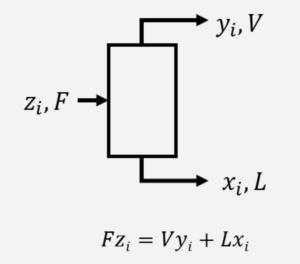LearnChemE

#### Material Balances: Screencasts

A general material balance is presented and simplifications of the balance are shown for different processes.

We suggest you list the important points in this screencast as a way to increase retention.

Reviews material balances using a flash system.

We suggest you list the important points in this screencast as a way to increase retention.

##### Important Equations:

$\stackrel{.}{m}_{in} = \stackrel{.}{m}_{out}$
where $$\stackrel{.}{m}_{in}$$ is the total mass flow into the system per unit time, and $$\stackrel{.}{m}_{out}$$ is the total mass flow out of the system per unit time. If a reaction is involved, the mass balance can be performed on individual atoms.where $$F$$, $$L$$, and $$V$$ are mass flow rates (kg/s) and $$z_{i}$$, $$x_{i}$$, and $$y_{i}$$ are mass fractions of component $$i$$ in each stream. A material balance can be done for each component.